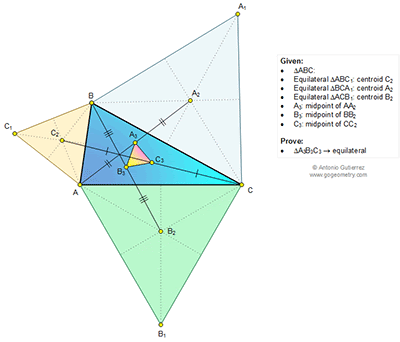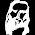## Saturday, June 20, 2015

### Geometry Problem 1125: Triangle, Four Equilateral Triangles, Centroid, Midpoint

Level: Mathematics Education, High School, Honors Geometry, College.

Click the diagram below to enlarge it.#### 1 comment:

1.Let ω = cis(2π/3). Let z(P) be the complex number representing P.

Consider
z(A₃) + ω z(B₃) + ω² z(C₃)
= 1/2 [z(A) + ω z(B) + ω² z(C)] + 1/2 [z(A₂) + ω z(B₂) + ω² z(C₂)]
= 1/6 {[z(A) + ω z(B) + ω² z(C)] + [z(A₁) + z(B) + z(C)] + ω[z(A) + z(B₁) + z(C)] + ω²[z(A) + z(B) + z(C₁)]}
= 1/6 {[z(A) + ω z(B) + ω² z(C₁)] + [z(A) + ω z(B₁) + ω² z(C)] + [z(A₁) + ω z(B) + ω² z(C)] + [1 + ω + ω²][z(A) + z(B) + z(C)]}
= 1/6 (0 + 0 + 0 + 0)
= 0

The result follows.